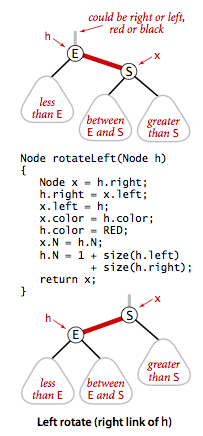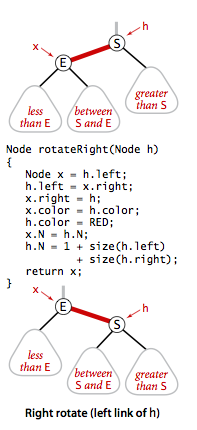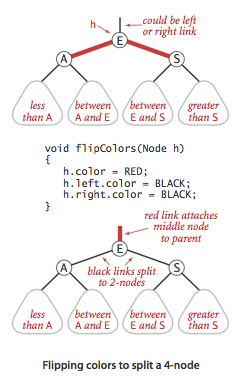# 左斜红黑树

Wafer Li ... 2017-02-21 《Algorithm 第四版》笔记
• Algorithm
• 读书笔记

## # 1. 介绍1. 红链接永远在左边（向左倾斜）
2. 一个节点不能同时链接两个红链接
3. 红黑树是完美黑链接平衡的

## # 2. 新的节点定义

``````pravite class Node {
Key key;
Value val;
int N;
boolean color;
}
``````
1
2
3
4
5
6

## # 3. 变形

### # 3.1 旋转

#### # 3.1.1 向左旋转1. 交换根节点
2. 将中间子树调换父亲

``````Node rotateLeft(Node h) {
Node x = h.right;
h.right = x.left;   // Link the middle
x.left = h;         // x ship to the root
x.color = h.color;  // Change color
h.color = RED;      // Change color
x.N = h.N;          // Ship amount

// Calculate the amount of left subtree
h.N = 1 + size(h.left) + size(h.right);

return x;           // Return new root
}
``````
1
2
3
4
5
6
7
8
9
10
11
12
13

#### # 3.1.2 向右旋转### # 3.2 颜色转换

1. 将两个红链接变成黑链接
2. 将父节点的链接颜色变为红色``````void filpColors(Node h) {
h.color = RED;
h.left.color = BLACK;
h.right.color = BLACK;
}
``````
1
2
3
4
5

## # 4. 插入

1. 新节点的颜色是红色的

由于 2-3 树在插入之后一定会形成至少一个 3-节点（有时还会有临时的 4-节点）

2. 如果右子结点是红色，左子结点是黑色，那么向左旋转

右子结点为红色，左子结点为黑色，说明红黑树中存在红色的右链接，将其向左旋转

3. 如果左子结点和它的左子结点都是红色的，那么将当前节点向右旋转

这种情况说明红黑树中存在两个连续的红色链接，说明存在一个内部的 4-节点，此时我们将其向右旋转，变为可以进行颜色转换的状态，随后通过颜色转换来将 4-节点 分裂

4. 如果左子结点和右子结点都是红色的，那么进行颜色转换

此时说明存在 4-节点，通过颜色转换将其分裂

``````public void put(Key key, Value val) {
root = put(root, key, val);
root.color = BLACK;
}

private Node put(Node h, Key key, Value val) {
if (h == null)
return new Node(key, val, 1, RED);

// 插入位置的搜寻过程
int cmp = key.compareTo(h.key);
if (cmp < 0)
h.left = put(h.left, key, val);
else if (cmp > 0)
h.right = put(h.right, key, val);
else
h.val = val;

// 旋转和颜色转换
// 注意以下顺序不可改变
if (isRed(h.right) && !isRed(h.left))
h = rotateLeft(h);

if (isRed(h.left) && isRed(h.left.left))
h = rotateRight(h);

if (isRed(h.left) && isRed(h.right))
flipColors(h);

h.N = size(h.left) + size(h.right) + 1;

return h;
}
``````
1
2
3
4
5
6
7
8
9
10
11
12
13
14
15
16
17
18
19
20
21
22
23
24
25
26
27
28
29
30
31
32
33## # 5. 删除

### # 5.1 删除最大最小元素

`b` 节点并不在我们的递归路线中，我们无法对这种非法的 4-节点进行修复；``````private Node moveRedLeft(Node h) {
//红链接向下传递
colorFlip(h);

// 出现红色后继
if (isRed(h.right.left)) {
h.right = rotateRight(h.right);
h = rotateLeft(h);
colorFlip(h);
}

return h;
}
``````
1
2
3
4
5
6
7
8
9
10
11
12
13

``````public void deleteMin() {
// 如果根节点的两个子节点都是黑色的，那么将根节点设为红
// 以求能够有红链接属性向下传递
if (!isRed(root.left) && !isRed(root.right))
root.color = RED;

root = deleteMin(root);

// 递归结束，将根节点恢复颜色
if (!isEmpty())
root.color = BLACK;
}

private Node deleteMin(Node h) {
if (h.left == null)
return null;

// 如果连续两个子节点都是黑链接，那么将红链接性质传递
if (!isRed(h.left) && !isRed(h.left.left))
h = moveRedLeft(h);

h.left = deleteMin(h.left);

// 递归向上修复链接性质
return balance(h);
}

// 实际上就是插入时使用的性质修复
private Node balance(Node h) {

if (isRed(h.right))
h = rotateLeft(h);
if (isRed(h.left) && isRed(h.left.left))
h = rotateRight(h);
if (isRed(h.left) && isRed(h.right))
flipColors(h);

h.size = size(h.left) + size(h.right) + 1;
return h;
}
``````
1
2
3
4
5
6
7
8
9
10
11
12
13
14
15
16
17
18
19
20
21
22
23
24
25
26
27
28
29
30
31
32
33
34
35
36
37
38
39
40``````private Node moveRedRight(Node h) {
// 红链接向下传递
flipColors(h);

// 出现连续左斜红色
if (isRed(h.left.left)) {
h = rotateRight(h);
flipColors(h);
}

return h;
}
``````
1
2
3
4
5
6
7
8
9
10
11
12

``````public void deleteMax() {
// 保证有红链接存在
if (!isRed(root.left) && !isRed(root.right))
root.color = RED;

root = deleteMax(root);

// 恢复根节点的黑链接
if (!isEmpty())
root.color = BLACK;
}

private Node deleteMax(Node h) {
// 由于是左斜红黑树，所以需要将左边的红链接右转
// 以能够向下传递
if (isRed(h.left))
h = rotateRight(h);

if (h.right == null)
return null;

// 如果没有连续的红链接，那么就将红链接向下传递
if (!isRed(h.right) && !isRed(h.right.left))
h = moveRedRight(h);

h.right = deleteMax(h.right);

return balance(h);
}
``````
1
2
3
4
5
6
7
8
9
10
11
12
13
14
15
16
17
18
19
20
21
22
23
24
25
26
27
28
29

### # 5.2 通常节点的删除

1. 将节点设置为其后继节点
2. 将其后继节点删除

``````public void delete(Key key) {
if (key == null)
throw new  IllegalArgumentException("argument to delete() is null");
if (!contains(key)) return;

// 保证红链接向下传递
if (!isRed(root.left) && !isRed(root.right))
root.color = RED;

root = delete(root, key);

// 递归结束，将根节点恢复
if (!isEmpty())
root.color = BLACK;
}

private Node delete(Node h, Key key) {
if (key.compareTo(h.key) < 0)  {    // 在左子树

// 如果没有连续的红链接，则将红链接向下传递
if (!isRed(h.left) && !isRed(h.left.left))
h = moveRedLeft(h);

h.left = delete(h.left, key);
}
else {  // 在右子树或者命中

// 左斜红黑树
// 将左边的红链接向右转，以向下传递
if (isRed(h.left))
h = rotateRight(h);

// 到达最大节点
if (key.compareTo(h.key) == 0 && (h.right == null))
return null;

// 没有连续的红链接，将红链接向下传递
if (!isRed(h.right) && !isRed(h.right.left))
h = moveRedRight(h);

// 内部节点
if (key.compareTo(h.key) == 0) {

// 后继
Node x = min(h.right);

// 将节点交换为后继
h.key = x.key;
h.val = x.val;

// 删除后继节点
h.right = deleteMin(h.right);
}
else
h.right = delete(h.right, key);
}
return balance(h);
}
``````
1
2
3
4
5
6
7
8
9
10
11
12
13
14
15
16
17
18
19
20
21
22
23
24
25
26
27
28
29
30
31
32
33
34
35
36
37
38
39
40
41
42
43
44
45
46
47
48
49
50
51
52
53
54
55
56
57
58